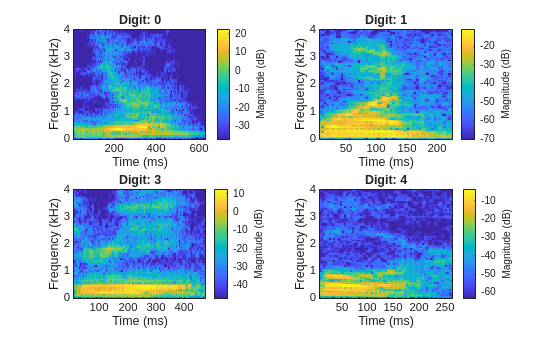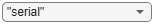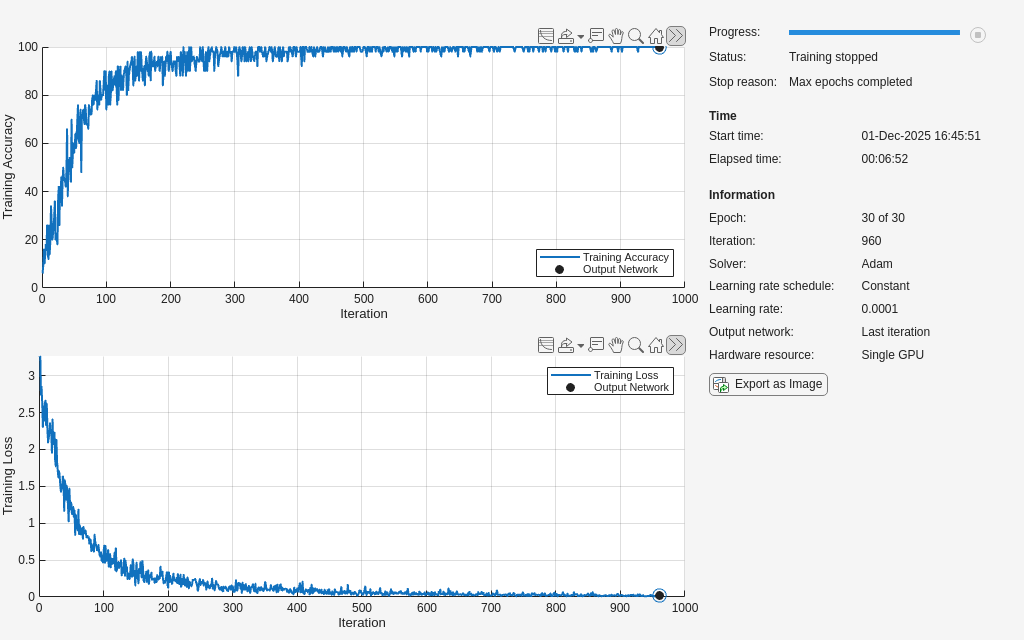# Spoken Digit Recognition with Custom Log Spectrogram Layer and Deep Learning

This example shows how to classify spoken digits using a deep convolutional neural network (CNN) and a custom log spectrogram layer. The custom layer uses the `dlstft` function to compute short-time Fourier transforms in a way that supports automatic back propagation.

### Data

Clone or download the Free Spoken Digit Dataset (FSDD), available at https://github.com/Jakobovski/free-spoken-digit-dataset. FSDD is an open data set, which means that it can grow over time. This example uses the version committed on August 12, 2020, which consists of 3000 recordings in English of the digits 0 through 9 obtained from six speakers. Each digit is spoken 50 times by each speaker. The data is sampled at 8000 Hz.

Use `audioDatastore` to manage data access. Set the `location` property to the location of the FSDD recordings folder on your computer. This example uses the base folder returned by MATLAB's `tempdir` command.

```pathToRecordingsFolder = fullfile(tempdir,'free-spoken-digit-dataset','recordings'); location = pathToRecordingsFolder; ads = audioDatastore(location);```

The helper function `helpergenLabels` creates a categorical array of labels from the FSDD files. The source code for `helpergenLabels` is listed in the appendix. List the classes and the number of examples in each class.

```ads.Labels = helpergenLabels(ads); summary(ads.Labels)```
``` 0 300 1 300 2 300 3 300 4 300 5 300 6 300 7 300 8 300 9 300 ```

Extract four audio files corresponding to different digits. Use `stft` to plot their spectrograms in decibels. Differences in the formant structure of the utterances are discernible in the spectrogram. This makes the spectrogram a reasonable signal representation for learning to distinguish the digits in a deep network.

```adsSample = subset(ads,[1,301,601,901]); SampleRate = 8000; for i = 1:4 [audioSamples,info] = read(adsSample); subplot(2,2,i) stft(audioSamples,SampleRate,'FrequencyRange','onesided'); title('Digit: '+string(info.Label)) end```Split the FSDD into training and test sets while maintaining equal label proportions in each subset. For reproducible results, set the random number generator to its default value. Eighty percent, or 2400 recordings, are used for training. The remaining 600 recordings, 20% of the total, are held out for testing.

```rng default; ads = shuffle(ads); [adsTrain,adsTest] = splitEachLabel(ads,0.8);```

Confirm that both the training and test sets contain the correct proportions of each class.

`disp(countEachLabel(adsTrain))`
``` Label Count _____ _____ 0 240 1 240 2 240 3 240 4 240 5 240 6 240 7 240 8 240 9 240 ```
`disp(countEachLabel(adsTest))`
``` Label Count _____ _____ 0 60 1 60 2 60 3 60 4 60 5 60 6 60 7 60 8 60 9 60 ```

The recordings in FSDD do not have a uniform length in samples. To use the spectrogram as the signal representation in a deep network, a uniform input length is required. An analysis of the audio recordings in this version of FSDD indicates that a common length of 8192 samples is appropriate to ensure that no spoken digit is cut off. Recordings greater than 8192 samples in length are truncated to 8192 samples, while recordings with fewer than 8192 samples are symmetrically padded to a length of 8192. The helper function `helperReadSPData` truncates or pads the data to 8192 samples and normalizes each recording by its maximum value. The source code for `helperReadSPData` is listed in the appendix. This helper function is applied to each recording by using a transform datastore in conjunction with `audioDatastore`.

```transTrain = transform(adsTrain,@(x,info)helperReadSPData(x,info),'IncludeInfo',true); transTest = transform(adsTest,@(x,info)helperReadSPData(x,info),'IncludeInfo',true);```

### Define Custom Log Spectrogram Layer

When any signal processing is done outside the network as pre-processing steps, there is a greater chance that network predictions are made with different pre-processing settings than those used in network training. This can have a significant impact on network performance, typically leading to poorer performance than expected. Placing the spectrogram or any other pre-processing computations inside the network as a layer gives you a self-contained model and simplifies the pipeline for deployment. It allows you to efficiently train, deploy, or share your network with all the required signal processing operations included. In this example, the chief signal processing operation is the computation of the spectrogram. The ability to compute the spectrogram inside the network is useful for both inference and when the device storage space is insufficient to save the spectrograms. Computing the spectrogram in the network only requires sufficient memory allocation for the current batch of spectrograms. However, it should be noted that this is not the optimal choice in terms of training speed. If you have sufficient memory, training time is significantly reduced by pre-computing all the spectrograms and storing those results. Then, to train the network, read the spectrogram "images" from storage instead of the raw audio and input the spectrograms directly in the network. Note that while this results in the fastest training time, the ability to perform signal processing inside the network still has considerable advantages for the reasons previously cited.

In training deep networks, it is often advantageous to use the logarithm of the signal representation because the logarithm acts like a dynamic range compressor, boosting representation values that have small magnitudes (amplitudes) but still carry important information. In this example, the log spectrogram performs better than the spectrogram. Accordingly, this example creates a custom log spectrogram layer and inserts it into the network after the input layer. Refer to Define Custom Deep Learning Layers (Deep Learning Toolbox) for more information about how to create a custom layer.

#### Declare the Parameters and Create Constructor Function

`logSpectrogramLayer` is a layer without learnable parameters, so only non-learnable properties are needed. Here the only required properties are those needed for spectrogram computation. Declare them in the `properties` section. In the layer's `predict` function, the `dlarray`-supported short-time Fourier transform function `dlstft` is used to compute the spectrogram. For more details on `dlstft` and these parameters, refer to the `dlstft` documentation. Create the function that constructs the layer and initializes the layer properties. Specify any variables required to create the layer as inputs to the constructor function.

```classdef logSpectrogramLayer < nnet.layer.Layer properties % (Optional) Layer properties. % Spectral window Window % Number of overlapped smaples OverlapLength % Number of DFT points FFTLength % Signal Length SignalLength end method function layer = logSpectrogramLayer(sigLength,NVargs) arguments sigLength {mustBeNumeric} NVargs.Window {mustBeFloat,mustBeNonempty,mustBeFinite,mustBeReal,mustBeVector}= hann(128,'periodic') NVargs.OverlapLength {mustBeNumeric} = 96 NVargs.FFTLength {mustBeNumeric} = 128 NVargs.Name string = "logspec" end layer.Type = 'logSpectrogram'; layer.Name = NVargs.Name; layer.SignalLength = sigLength; layer.Window = NVargs.Window; layer.OverlapLength = NVargs.OverlapLength; layer.FFTLength = NVargs.FFTLength; end ... end ```

#### Predict Function

As previously mentioned, the custom layer uses `dlstft` to obtain the STFT and then computes the logarithm of the squared magnitude STFT to obtain log spectrograms. You can also remove the `log` function if you wish or add any other dlarray-supported function to customize the output. You can copy `logSpectrogramLayer.m` to a different folder if you want to experiment with different outputs from the predict function. It is recommended to save the custom layer under a different name to prevent any conflicts with the version used in this example.

``` function Z = predict(layer, X) % Forward input data through the layer at prediction time and % output the result. % % Inputs: % layer - Layer to forward propagate through % X - Input data, specified as a 1-by-1-by-C-by-N % dlarray, where N is the mini-batch size. % Outputs: % Z - Output of layer forward function returned as % an sz(1)-by-sz(2)-by-sz(3)-by-N dlarray, % where sz is the layer output size and N is % the mini-batch size. % Use dlstft to compute short-time Fourier transform. % Specify the data format as SSCB to match the output of % imageInputLayer. X = squeeze(X); [YR,YI] = dlstft(X,'Window',layer.Window,... 'FFTLength',layer.FFTLength,'OverlapLength',layer.OverlapLength,... 'DataFormat','TBC'); % This code is needed to handle the fact that 2D convolutional % DAG networks expect SSCB YR = permute(YR,[1 4 2 3]); YI = permute(YI,[1 4 2 3]); % Take the logarithmic squared magnitude of short-time Fourier % transform. Z = log(YR.^2 + YI.^2); end ```

Because logSpectrogramLayer uses the same forward pass for training and prediction (inference), only the `predict` function is needed and no `forward` function is required. Additionally, because the `predict` function uses `dlstft`, which supports `dlarray`, differentiation in backward propagation can be done automatically. This means that you do not have to write a `backward` function. This is a significant advantage in writing a custom layer that supports `dlarray`. For a list of functions that support `dlarray` objects, see List of Functions with dlarray Support (Deep Learning Toolbox).

### Deep Convolutional Neural Network (DCNN) Architecture

You can use a custom layer in the same way as any other layer in Deep Learning Toolbox. Construct a small DCNN as a layer array that includes the custom layer `logSpectrogramLayer`. Use convolutional and batch normalization layers and downsample the feature maps using max pooling layers. To guard against overfitting, add a small amount of dropout to the input of the last fully connected layer.

```sigLength = 8192; dropoutProb = 0.2; numF = 12; layers = [ imageInputLayer([sigLength 1]) logSpectrogramLayer(sigLength,'Window',hamming(1280),'FFTLength',1280,... 'OverlapLength',900) convolution2dLayer(5,numF,'Padding','same') batchNormalizationLayer reluLayer maxPooling2dLayer(3,'Stride',2,'Padding','same') convolution2dLayer(3,2*numF,'Padding','same') batchNormalizationLayer reluLayer maxPooling2dLayer(3,'Stride',2,'Padding','same') convolution2dLayer(3,4*numF,'Padding','same') batchNormalizationLayer reluLayer maxPooling2dLayer(3,'Stride',2,'Padding','same') convolution2dLayer(3,4*numF,'Padding','same') batchNormalizationLayer reluLayer convolution2dLayer(3,4*numF,'Padding','same') batchNormalizationLayer reluLayer maxPooling2dLayer(2) dropoutLayer(dropoutProb) fullyConnectedLayer(numel(categories(ads.Labels))) softmaxLayer classificationLayer('Classes',categories(ads.Labels)); ];```

Set the hyperparameters to use in training the network. Use a mini-batch size of `50` and a learning rate of `1e-4`. Specify Adam optimization. Set `UsePrefetch `to `true `to enable asynchronous prefetch and queuing of data to optimize training performance. Background dispatching of data and using a GPU to train the network requires Parallel Computing Toolbox™.

```UsePrefetch =true; options = trainingOptions('adam', ... 'InitialLearnRate',1e-4, ... 'MaxEpochs',30, ... 'MiniBatchSize',50, ... 'Shuffle','every-epoch', ... 'DispatchInBackground',UsePrefetch,... 'Plots','training-progress',... "Verbose",false);```

Train the network.

`[trainedNet,trainInfo] = trainNetwork(transTrain,layers,options);`Use the trained network to predict the digit labels for the test set. Compute the prediction accuracy.

```[YPred,probs] = classify(trainedNet,transTest); cnnAccuracy = sum(YPred==adsTest.Labels)/numel(YPred)*100```
```cnnAccuracy = 97 ```

Summarize the performance of the trained network on the test set with a confusion chart. Display the precision and recall for each class by using column and row summaries. The table at the bottom of the confusion chart shows the precision values. The table to the right of the confusion chart shows the recall values.

```figure('Units','normalized','Position',[0.2 0.2 0.5 0.5]); ccDCNN = confusionchart(adsTest.Labels,YPred); ccDCNN.Title = 'Confusion Chart for DCNN'; ccDCNN.ColumnSummary = 'column-normalized'; ccDCNN.RowSummary = 'row-normalized';```### Summary

This example showed how to create a custom spectrogram layer using `dlstft`. Using functionality that supports `dlarray`, the example demonstrated how to embed the signal processing operations inside the network in a way which supports backpropagation and the use of GPUs.

### Appendix: Helper Functions

```function Labels = helpergenLabels(ads) % This function is only for use in the "Spoken Digit Recognition with % Custom Log Spectrogram Layer and Deep Learning" example. It may change or % be removed in a future release. tmp = cell(numel(ads.Files),1); expression = "[0-9]+_"; for nf = 1:numel(ads.Files) idx = regexp(ads.Files{nf},expression); tmp{nf} = ads.Files{nf}(idx); end Labels = categorical(tmp); end```
```function [out,info] = helperReadSPData(x,info) % This function is only for use in the "Spoken Digit Recognition with % Custom Log Spectrogram Layer and Deep Learning" example. It may change or % be removed in a future release. N = numel(x); if N > 8192 x = x(1:8192); elseif N < 8192 pad = 8192-N; prepad = floor(pad/2); postpad = ceil(pad/2); x = [zeros(prepad,1) ; x ; zeros(postpad,1)]; end x = x./max(abs(x)); out = {x./max(abs(x)),info.Label}; end```